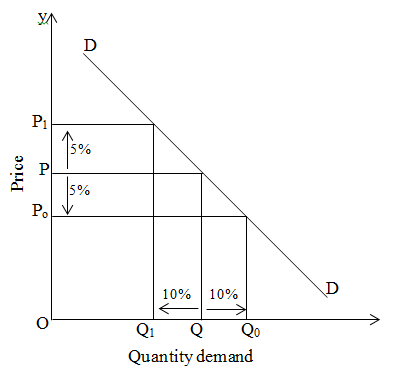# Relatively elastic demand

If the percentage change in quantity demand is greater than the percentage change in price is known as relatively elastic demand. For example 10% change in demand due to 5% change in demand; we can explain it by following figureOn the above figure, when price is OP then quantity demand for that commodity is OQ. When prices become P1 by increasing by 5% then quantity demand decreases from Q to Q1 by 10% and when price decreases by 5% to Po then quantity demand increases from Q to Qo by 10%.